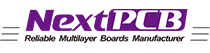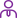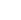### New Life with Your NEXTPCB!

Turnkey PCB manufacturing & assembly services.

LanguageLogin|Sign up
Blog >> Blog Details Page
Calculation of characteristic impedance
Posted:03:33 PM July 16, 2018 writer: G

The impedance Z seen by the signal in each propagation interval is consistent with the basic definition of impedance.

Z=V/I

Here, the voltage V refers to the signal voltage applied to the transmission line, and the current I refers to the total amount of charge δQ obtained from the battery during each time interval δt, so

I=δQ/δt

The charge flowing into the transmission line (these charges ultimately come from the signal source) is used to charge the capacitance δC formed between the signal line and the return path added during the propagation to the voltage V, so

δQ=VδC

We can relate the capacitance caused by the signal to a certain distance during propagation to the capacitance value CL over the unit length of the transmission line and the speed U at which the signal propagates on the transmission line. At the same time, the distance traveled by the signal is the velocity U multiplied by the time interval δt. and so

δC= CL U δt

Combining all of the above equations, we can deduce that the instantaneous impedance is:

Z=V/I=V/(δQ/δt)=V/(VδC/δt)=V/(V CL U δt /δt)=1/(CL U)

It can be seen that the instantaneous impedance is related to the capacitance value on the length of the unit transmission line and the speed of signal transmission. It can also be artificially defined as the characteristic impedance of the transmission line. In order to distinguish the characteristic impedance from the actual impedance Z, a subscript 0 is deliberately added to the characteristic impedance. From the above derivation, the characteristic impedance of the signal transmission line has been obtained:

Z0=1/(CL U)

If the capacitance value per unit length on the transmission line and the speed at which the signal propagates on the transmission line are kept constant, then the transmission line has a constant characteristic impedance over its length, and such a transmission line is referred to as a transmission line of controlled impedance.

As can be seen from the above brief description, some intuitive understanding of capacitance can be linked to the intuitive understanding of the newly discovered characteristic impedance. In other words, if the signal wiring in the PCB is widened, the capacitance value per unit length of the transmission line is increased, and the characteristic impedance of the transmission line can be lowered.

Intriguing topic

Some confusion about the characteristic impedance of the transmission line can often be heard. According to the above analysis, after connecting the signal source to the transmission line, you should be able to see the characteristic impedance of the transmission line of a certain value, for example 50Ω. However, if an ohmmeter is connected to the same 3 foot long RG58 cable, The measured impedance is infinite.

The answer to the question is that the impedance values seen from the front end of any transmission line change over time. If the time to measure the impedance of the cable is as short as the time it takes for the signal to travel back and forth in the cable, you can measure the surge impedance of the cable or the characteristic impedance of the cable. However, if you wait for enough time, some of the energy will be reflected back and detected by the measuring instrument. At this time, the impedance change can be detected. Usually, in this process, the impedance will change back and forth until the impedance value. A stable state is reached: If the end of the cable is open, the final impedance is infinite. If the end of the cable is shorted, the final impedance is zero.

For a 3 foot long RG58 cable, the impedance measurement process must be completed in less than 3 ns intervals. This is what the Time Domain Reflectometry (TDR) is doing. The TDR measures the dynamic impedance of the transmission line. If you need to spend 1s of time to measure the impedance of a 3 foot long RG58 cable, then the signal has been reflected back and forth millions of times during this time interval, then you may get completely different from the huge changes in impedance. The value of the impedance, the final result is infinity, because the end of the cable is open.

517 1 0 1
• PCB
Portotype
• PCB
Assembly
• SMD
Stencil
 Dimensions: (mm) × Quantity: (pcs) 5 5 10 15 20 25 30 40 50 75 100 120 150 200 250 300 350 400 450 500 600 700 800 900 1000 1500 2000 2500 3000 3500 4000 4500 5000 5500 6000 6500 7000 7500 8000 9000 10000 Other Quantities:(quantity*length*width is greater than 10㎡) OK Layers: 1 2 4 6 8 10 12 Thickness: 0.6 mm 0.8 mm 1.0 mm 1.2 mm 1.6 mm 2.0 mm 2.5 mmQuote now